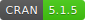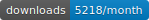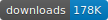Release:## Zelig workflow overview

All models in Zelig can be estimated and results explored presented using four simple functions:

1. `zelig` to estimate the parameters,

2. `setx` to set fitted values for which we want to find quantities of interest,

3. `sim` to simulate the quantities of interest,

4. `plot` to plot the simulation results.

#### Zelig 5 reference classes

Zelig 5 introduced reference classes. These enable a different way of working with Zelig that is detailed in a separate vignette. Directly using the reference class architecture is optional. They are not used in the examples below.

## Zelig Quickstart Guide

Let’s walk through an example. This example uses the swiss dataset. It contains data on fertility and socioeconomic factors in Switzerland’s 47 French-speaking provinces in 1888 (Mosteller and Tukey, 1977, 549-551). We will model the effect of education on fertility, where education is measured as the percent of draftees with education beyond primary school and fertility is measured using the common standardized fertility measure (see Muehlenbein (2010, 80-81) for details).

If you haven’t already done so, open your R console and install Zelig. We recommend installing Zelig with the zeligverse package. This installs core Zelig and ancillary packages at once.

``install.packages('zeligverse')``

Alternatively you can install the development version of Zelig with:

``devtools::install_github('IQSS/Zelig')``

Once Zelig is installed, load it:

``library(zeligverse)``

## Building Models

Let’s assume we want to estimate the effect of education on fertility. Since fertility is a continuous variable, least squares (`ls`) is an appropriate model choice. To estimate our model, we call the `zelig()` function with three two arguments: equation, model type, and data:

``````# load data
data(swiss)

# estimate ls model
z5_1 <- zelig(Fertility ~ Education, model = "ls", data = swiss, cite = FALSE)

# model summary
summary(z5_1)

## Model:
##
## Call:
## z5\$zelig(formula = Fertility ~ Education, data = swiss)
##
## Residuals:
##     Min      1Q  Median      3Q     Max
## -17.036  -6.711  -1.011   9.526  19.689
##
## Coefficients:
##             Estimate Std. Error t value Pr(>|t|)
## (Intercept)  79.6101     2.1041  37.836  < 2e-16
## Education    -0.8624     0.1448  -5.954 3.66e-07
##
## Residual standard error: 9.446 on 45 degrees of freedom
## Multiple R-squared:  0.4406, Adjusted R-squared:  0.4282
## F-statistic: 35.45 on 1 and 45 DF,  p-value: 3.659e-07
##
## Next step: Use 'setx' method``````

The -0.86 coefficient on education suggests a negative relationship between the education of a province and its fertility rate. More precisely, for every one percent increase in draftees educated beyond primary school, the fertility rate of the province decreases 0.86 units. To help us better interpret this finding, we may want other quantities of interest, such as expected values or first differences. Zelig makes this simple by automating the translation of model estimates into interpretable quantities of interest using Monte Carlo simulation methods (see King, Tomz, and Wittenberg (2000) for more information). For example, let’s say we want to examine the effect of increasing the percent of draftees educated from 5 to 15. To do so, we set our predictor value using the `setx()` and `setx1()` functions:

``````# set education to 5 and 15
z5_1 <- setx(z5_1, Education = 5)
z5_1 <- setx1(z5_1, Education = 15)

# model summary
summary(z5_1)

## setx:
##   (Intercept) Education
## 1           1         5
## setx1:
##   (Intercept) Education
## 1           1        15
##
## Next step: Use 'sim' method``````

After setting our predictor value, we simulate using the `sim()` method:

``````# run simulations and estimate quantities of interest
z5_1 <- sim(z5_1)

# model summary
summary(z5_1)

##
##  sim x :
##  -----
## ev
##       mean       sd      50%     2.5%    97.5%
## 1 75.30616 1.658283 75.28057 72.12486 78.48007
## pv
##          mean       sd      50%     2.5%   97.5%
## [1,] 75.28028 9.707597 75.60282 57.11199 94.3199
##
##  sim x1 :
##  -----
## ev
##       mean       sd      50%     2.5%    97.5%
## 1 66.66467 1.515977 66.63699 63.66668 69.64761
## pv
##          mean       sd      50%     2.5%    97.5%
## [1,] 66.02916 9.441273 66.32583 47.19223 82.98039
## fd
##        mean       sd       50%      2.5%     97.5%
## 1 -8.641488 1.442774 -8.656953 -11.43863 -5.898305``````

At this point, we’ve estimated a model, set the predictor value, and estimated easily interpretable quantities of interest. The `summary()` method shows us our quantities of interest, namely, our expected and predicted values at each level of education, as well as our first differences–the difference in expected values at the set levels of education.

# Visualizations

Zelig’s `plot()` function plots the estimated quantities of interest:

``plot(z5_1)``

We can also simulate and plot simulations from ranges of simulated values:

``````z5_2 <- zelig(Fertility ~ Education, model = "ls", data = swiss, cite = FALSE)

# set Education to range from 5 to 15 at single integer increments
z5_2 <- setx(z5_2, Education = 5:15)

# run simulations and estimate quantities of interest
z5_2 <- sim(z5_2)``````

Then use the `plot()` function as before:

``z5_2 <- plot(z5_2)``

# Getting help

The primary documentation for Zelig is available at: http://docs.zeligproject.org/articles/.

Within R, you can access function help using the normal `?` function, e.g.:

``?setx``

If you are looking for details on particular estimation model methods, you can also use the `?` function. Simply place a `z` before the model name. For example, to access details about the `logit` model use:

``?zlogit``

# Building Zelig (for developers)

Zelig can be fully checked and build using the code in check_build_zelig.R. Note that this can be time consuming due to the extensive test coverage.